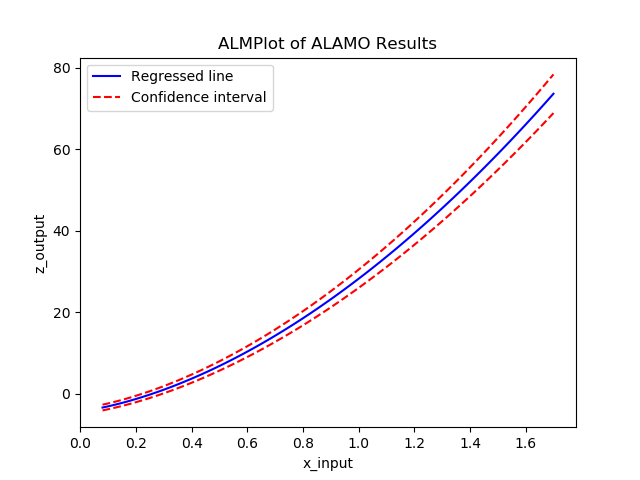# ALAMOPY : ALAMO Python¶

The purpose of ALAMOPY (Automatic Learning of Algebraic MOdels PYthon wrapper) is to provide a wrapper for the software ALAMO which generates algebraic surrogate models of black-box systems for which a simulator or experimental setup is available. Consider a system for which the outputs z are an unknown function f of the system inputs x. The software identifies a function f, i.e., a relationship between the inputs and outputs of the system, that best matches data (pairs of x and corresponding z values) that are collected via simulation or experimentation.

## Basic Usage¶

ALAMOPY’s main function is alamopy.alamo. Data can be read in or simulated using available python packages. The main arguments of the alamopy.alamo python function are inputs and outputs, which are 2D arrays of data. For example

regression_results =alamopy.alamo(x_inputs, z_outputs, **kargs)


where **kargs is a set of named keyword arguments than can be passed to the alamo python function to customize the basis function set, names of output files, and other options available in ALAMO.

Warning

The alamopy.doalamo function is deprecated. It is being replaced with alamopy.alamo

### Options for alamopy.alamo¶

Possible arguments to be passed to ALAMO through do alamo and additional arguments that govern the behavior of doalamo.

• xlabels - list of strings to label the input variables
• zlabels - list of strings to label the output variables
• functions - logfcns, expfcns, cosfcns, sinfcns, linfcns, intercept. These are ‘0-1’ options to activate these functions
• monomialpower, multi2power, multi3power, ratiopower. List of terms to be used in the respective basis functions
• modeler - integer 1-7 determines the choice of fitness metrice
• solvemip - ‘0-1’ option that will force the solving of the .gms file

These options are specific to alamopy and will not change the behavior of the underlying .alm file.

• expandoutput - ‘0-1’ option that can be used to collect more information from the ALAMO .lst and .trc file
• showalm - ‘0-1’ option that controlif the ALAMO output is printed to screen
• almname - A string that will assign the name of the .alm file
• outkeys - ‘0-1’ option for dictionary indexing according to the output labels
• outkeys - ‘0-1’ option for dictionary indexing according to the output labels
• outkeys - ‘0-1’ option for dictionary indexing according to the output labels
• savetrace - ‘0-1’ option that controls the status of the trace file
• savescratch - ‘0-1’ option to save the .alm and .lst files
• almopt - A string option that will append a text file of the same name to the end of each .alm fille to faciliate advanced user access in an automated fashion

## ALAMOPY Output¶

There are mutliple outputs from the running alamopy.alamo. Outputs include:

• f(model): A callable function
• pymodel: name of the python model written
• model: string of the regressed model

Note: A python script named after the output variables is written to the current directory. The model can be imported and used for further evaluation, for example to evaluate residuals:

import z1
residuals = [y-z1.f(inputs,inputs) for y,inputs in zip(z,x)]


After the regression of a model, ALAMOPY provides confidence interval analysis and plotting capabilities using the results output.

Plotting

The plotting capabilities of ALAMOPY are available in the almplot function. Almplot will plot the function based on one of the inputs.

result = alamopy.alamo(x_in, z_out, kargs)
alamopy.almplot(result)


Confidence intervals

Confidence intervals can similarly be calculated for the weighting of selected basis functions using the almconfidence function.

This adds conf_inv (confidence intervals) and covariance (covariance matrix) to the results dictionary. This also gets incorporated into the plotting function if it is available.

result = alamopy.alamo(x_in, z_out, kargs)
result = alamopy.almconfidence(result)
alamopy.almplot(result)Similar to ALAMO, there are advanced capabilities for customization and constrained regression facilitated by methods in ALAMOPY including custom basis functions, custom constraints on the response surface, and basis function groups. These methods interact with the regression using the alamo option file.

Custom Basis Functions

Custom basis functions can be added to the built-in functions to expand the functional forms available. To use this advanced capability in ALAMOPY, the following function is called. Note it is necessary to use the xlabels assigned to the input parameters.

addCustomFunctions(fcn_list)
addCustomFunctions(["x1^2 * x2^2", "...", "..." ...])


Custom Constraints

Custom constraints can be placed on response surface or regressed function of the output variable. In ALAMO, this is controlled using custom constraints, CUSTOMCON. The constraints, a function g(x_inputs, z_outputs) are applied to a specific output variable, which is the index of the output variable, and are less than or equal to 0 (g <= 0).

To use this advanced capability in ALAMOPY, the following function is called. Note it is necessary to use the xlabels assigned to the input parameters.

addCustomConstraints(custom_constraint_list, **kargs)
addCustomConstraints(["1 z1 - x1 + x2 +1", "...", "..." ...])


Basis Function Groups and Constraints

In addition to imposing constraints on the response surface it produces, ALAMO has the ability to enforce constraints on groups of selected basis functions. To define groups in ALAMOPY, you can use the following methods. Each Basis group has an index number that will be used as reference in the group constraints. The groups are defined by three or four parameters. Options for Member-type are LIN, LOG, EXP, SIN, COS, MONO, MULTI2, MULTI3, RATIO, GRP, RBF, and CUST.

addBasisGroup(type_of_function, input_indices, powers)



With the groups defined, constraints can be placed on the groups using the constraint-types NMT (no-more-than), ATL (at-least), REQ (requires), and XCL (exclude). For NMT and ATL the integer-parameter is the number of members in the group that should be selected based on the constraint. For REQ and XCL the integer-parameter is the group-id number of excluded or required basis functions.

To add the basis constraints to alamopy, you can use the following methods.

addBasisConstraint(group_id, output_id, constraint_type, intParam)